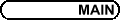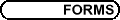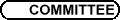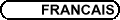Plenary Speakers / Conférenciers principaux MICHAEL BENNETT, University of British Columbia, Vancouver, BC Classical Diophantine equations via modern and not-so-modern methods In this talk, I will attempt to survey some recent developments in the field of Diophantine equations, with particular emphasis on a number of classical problems that have been resolved in the past few years, such as Catalan's Conjecture. Of particular interest are new techniques, such as those arising from the modularity of Galois representations. I will conclude with a list of what I hope are challenging open problems in this area. PERSI DIACONIS, Stanford A Mathematician Flips a Coin In joint work with Susan Holmes and Richard Montgomery we have proved that naturally flipped coins are biased to come up the same way they started. The analysis is a mix of mechanics and Tauberian Theorems coupled with image analysis and new statistical techniques for high-speed slow motion cameras. ROSTISLAV GRIGORCHUK, Texas A&M University Algebraic, algorithmic, and spectral properties of automata groups Automata groups were defined 40 years ago and started to play an important role two decades later when it was discovered that they provide solutions to such famous problems as Burnside problem on torsion groups or Milnor problem on growth of finitely generated groups. The development of the theory of automata groups during the last two decades showed that the number of difficult problems to which the methods and the ideas involving groups generated by finite automata can be applied is rather large and that they could be used in many areas of mathematics such as algebra, analysis, geometry, probability, dynamics, computer science, mathematical logic, and other. Automata groups were used to solve several difficult problems in analysis centered around the notion of amenability introduced by von Neumann in 1929 (as a result of his study of the algebraic roots behind the Banach-Tarski paradox), in Riemannian geometry (Atiyah Conjecture on L2 invariants), in theory of profinite groups (Zelmanov Conjecture on groups of finite width), etc. There are indications that automata groups can be used to attack Kaplansky-Kadison Conjecture on Idempotents, Kaplansky Conjecture on Jacobson radical, Dixmier Unitarizibility Problem, Baum-Connes and Novikov Conjectures, Fontaine-Mazur Conjecture in Number theory and other problems in mathematics. We will try to convince the audience that this is indeed the case. FRANÇOIS LALONDE, CRM How pure mathematical aspects of String theory can solve deep problems of geometric group theory In any simple group, the subgroup consisting of finite products of commutators must obviously be either the identity or the whole group. Thus, in a non-abelian simple group, any element is the product of a finite number of commutators. Said crudely: in such a group, the lack of commutativity is enough to generate the whole group! The "commutator length" of an element is the minimal number of commutators in such a product. It turns out that persistent efforts of topologists in the '70s and '80s led to the following theorem: the group of area preserving transformations of the 2-sphere (which is obviously non-abelian) is simple! (This is the simplest example of a much broader class that includes all algebraic manifolds and much more.) The methods used in this theorem are "soft", and give no idea on the behaviour of the commutator length of a given transformation (with respect, say, to iterations of this transformation). On the other hand, a simple non-abelian group has no non-trivial homomorphism to the reals (or to any abelian group). But there might be quasi-morphisms (maps that are almost homomorphisms) and I will explain how to construct such quasi-morphims, according to ideas of collaborators in Israel (Entov, Polterovich, Biran) that are based on a clever use of the semi-simplicity of quantum homology, i.e., on ideas based on Floer and String theories. I will then explain how these quasi-morphisms give the first significant informations on the commutator length problem. RAINER STEINWANDT, Universität Karlsruhe, 76131 Karlsruhe, Germany Non-abelian groups in public key cryptography While finite cyclic groups are a well-established tool in public key cryptography, there are not many practical proposals for cryptographic schemes based on non-abelian groups. One interesting line of research in this context, which has received a lot of attention in the cryptographic community, explores the cryptographic potential of braid groups. Other conceptually interesting proposals rely on "wild" factorizations of finite non-abelian groups and inspired (not only cryptographic) questions about the existence of certain factorizations of finite groups. The talk surveys some cryptographic schemes based on finite or finitely presented non-abelian groups and discusses some (non-)mathematical issues that have to be dealt with when trying to implement practical cryptographic schemes on the basis of non-abelian groups.